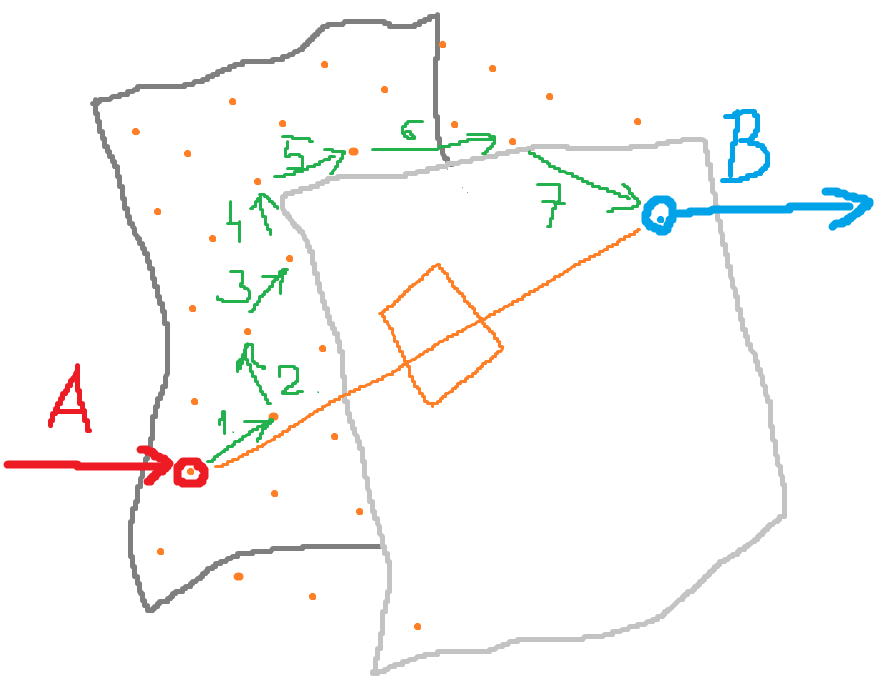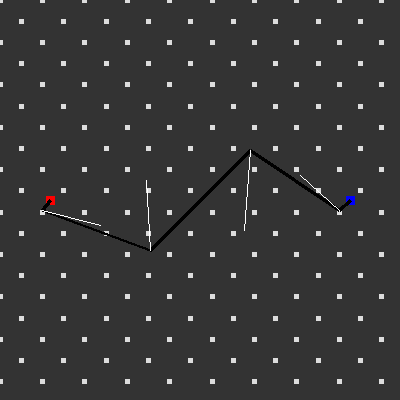# HomeOffice: path generation problem

Hi,

i got a problem here, where i would like to hear your opinions:

I got a bounding box closed by freeform surfaces. There is 2 point on the adjacent boundaries, called A and B.
I can create a uniform point-cloud in this bounding box, where the distance between any 2 neighbour point is T.
I need to find the straightest path between A and B. Of course a straight line between A and B would be the ideal, but thats not always possible.

Finding a path is just jumping from point to a neighbour point randomly and storing the point ID-s along the way.
Lets say Y jump is allowed, then an algorith can generate all the paths in matter of seconds.

I can close out all the paths which doesn’t ends in B.
Then we have all the paths, which starts in A and ends in B.

We know the allowed maximal path lenght (L), and as we know the count of jumps on every path and the distance between the points, we can close out all the paths which is longer.
Now we have a handful of paths which starts in A and ends in B and their lenghts is less than the allowed maximal lenght (L).

Now i am interested in the most straight path. This is tricky.
We can create a straight line between A and B. We can create a perpendicualr plane on this straight line.
Projecting the points of all the different paths on this plane results a planar point-cloud. The bigger the resulting point cloud the bigger the path deviance from a straight line (as each point of a straight line projected onto a plane perpendicular to the same line results points in the same place, technically one result).

Now this would result the straightest path between A and B which is shorter than the maximal lenght.Of course, one could implement the checks in the path-generating process, but i even my way would result solution in matter of minutes on modern hw.

I am not doing too much programming, but i have some experiences with Python. Is it possible to doing this with it?

Feel free to express constructive critique. Thank You!

A* path finding algorithm is your friend …

1 Like

I do not quite get the details of your constraint, but usually this kind of things are done via dynamic programming. That is you do not generate all the paths (which are too many) but you work backwards from the endpoint. Let’s say you have N steps. Then V_{N-1} is the function on the set of points which is dist(x,B) on the point which you can connect to B with one step and +infinity elsewhere. Then you define V_{N-2} to be the function which on point x has value min{dist(x,y)+V_{N-1}(y)} where y is any point you can connect to x in one step. And you go on to define all the other V_n. backwards. Now you start from A and jump to the point where V_1 is minimal, and then to the point where V_2 is minimal, etc… forward.

you need a metric to measure the straightness of your paths. I think of taking 3 consecutive points, calculate the real mid point and measure distance betwen the middle and this calculated one. You can then do some statistics with the result (averages, total, sd…).

In this picture i show this distance to the calculated mid point as white linesedit: you may also calculate a distance to some point using longer segments of your paths